In this example, we will describe how to use splines to model nonlinearities in predictor variables and the generalized additive model.

## Splines

Splines are nothing more than a set of linear or non-linear functions that are tied together to construct a model for data.

### piece-wise splines

In the simplest example, consider a regression function whose form changes at some point in the data. So if we see data like this:

b0<-1; b1=.5;b2=35
x<-seq(1:100)
y1<-rnorm(70,b0+b1*x[1:70], 1)
y2<-rnorm(30, b2, 1)

y<-c(y1, y2)
plot(y~x, main="linear fit to nonlinear data")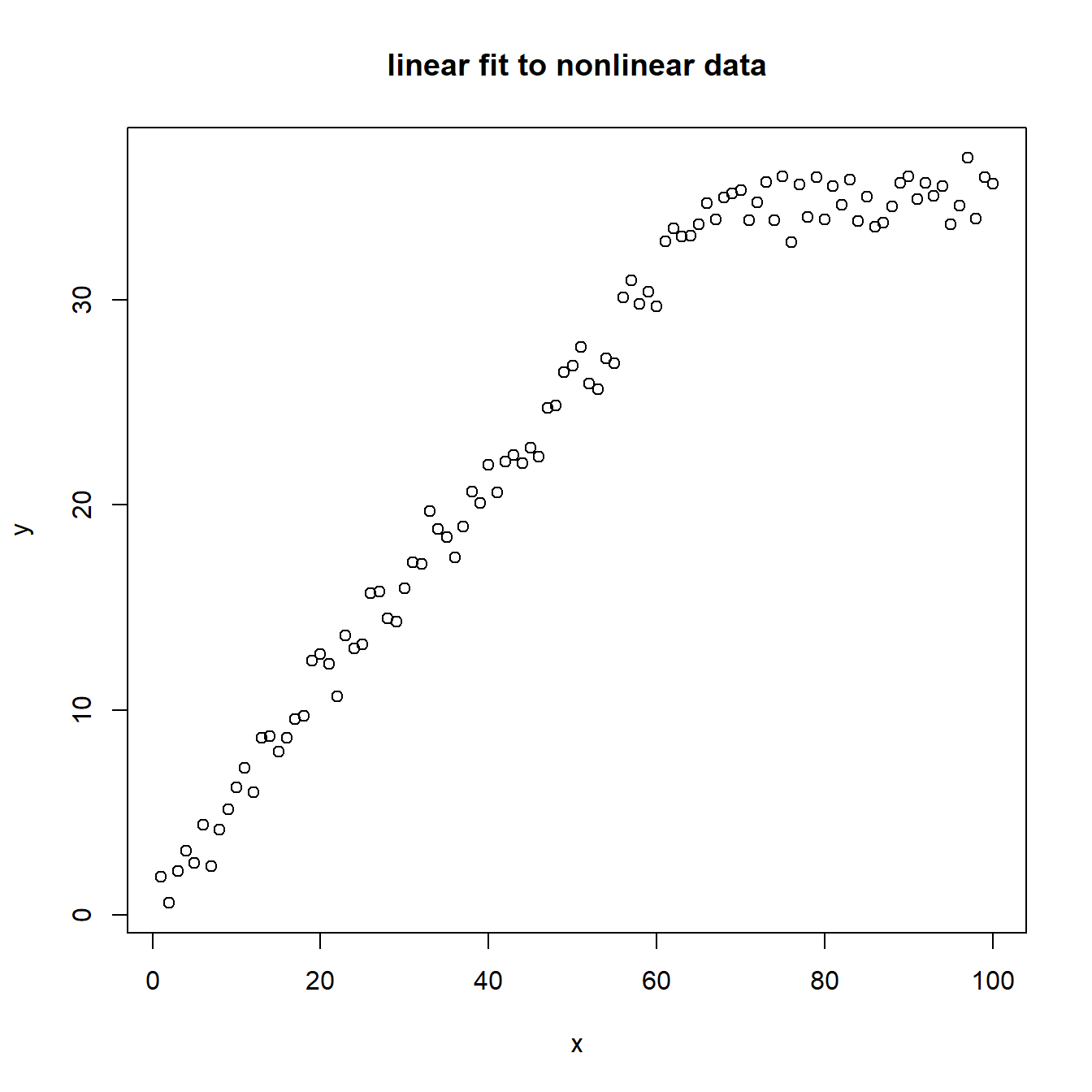we might say, yes, I can fit a linear model to that:

plot(y~x, main="linear fit to nonlinear data")
lin<-lm(y~x)
summary(lin)
##
## Call:
## lm(formula = y ~ x)
##
## Residuals:
##     Min      1Q  Median      3Q     Max
## -7.5338 -2.3689  0.4286  2.2613  5.5451
##
## Coefficients:
##             Estimate Std. Error t value Pr(>|t|)
## (Intercept)  4.59458    0.63262   7.263 9.14e-11 ***
## x            0.37634    0.01088  34.604  < 2e-16 ***
## ---
## Signif. codes:  0 '***' 0.001 '**' 0.01 '*' 0.05 '.' 0.1 ' ' 1
##
## Residual standard error: 3.139 on 98 degrees of freedom
## Multiple R-squared:  0.9243, Adjusted R-squared:  0.9236
## F-statistic:  1197 on 1 and 98 DF,  p-value: < 2.2e-16
lines(fitted(lin)~x,col=3)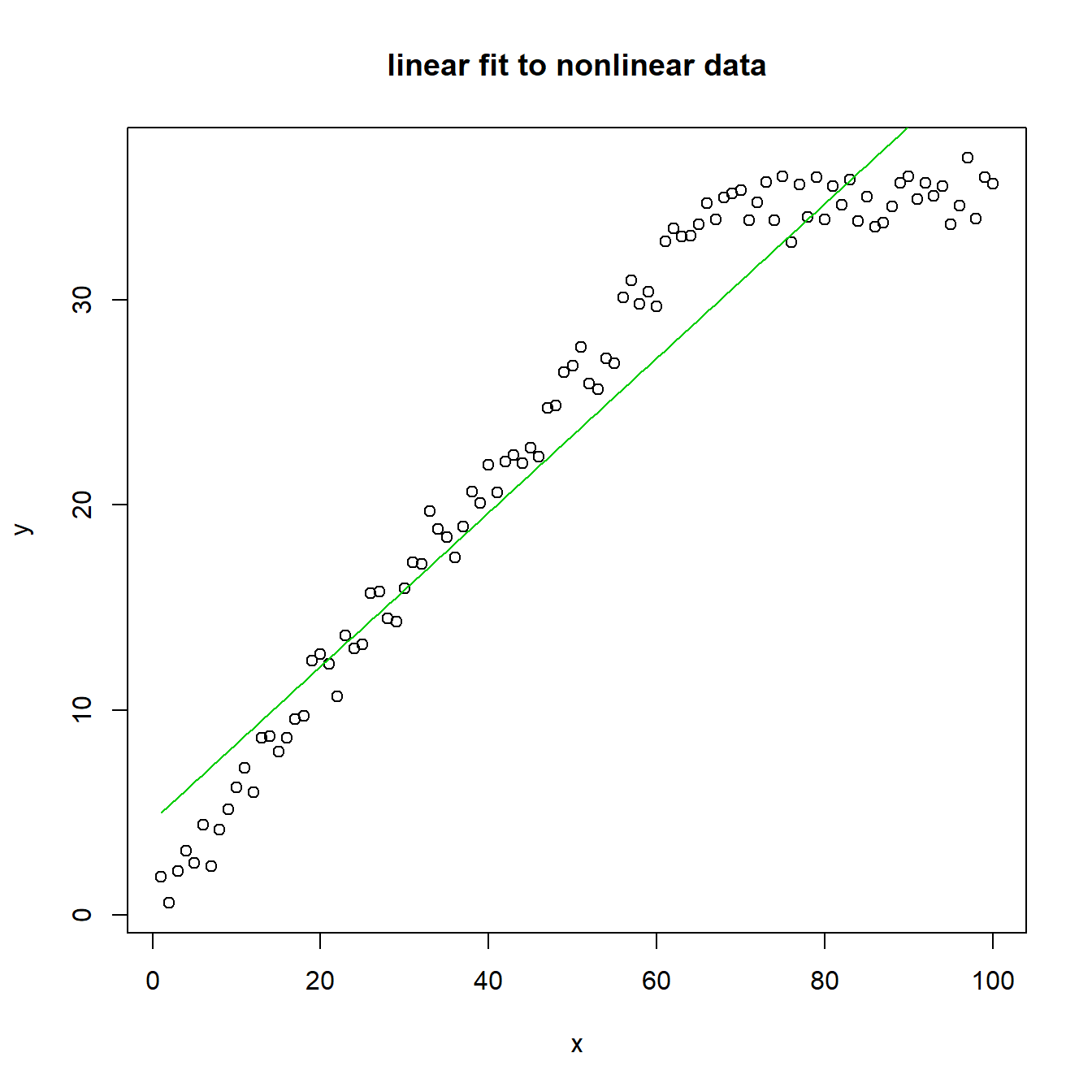but we would be sorely mistaken, as this model clearly doesn’t fit the data.

Instead, we can model the change in the underlying model structure by using a series of knots in the function, or points along the data in which the form of the function changes.

Mathematically, knots can be written as:

$$Y(x) = $\begin{Bmatrix} F_1(x) \text { for } x\in [x_1, x_2]\\ F_2(x) \text { for } x\in [x_2, x_3]\\ \cdots \\ F_{k}(x) \text { for } x\in [x_{k-1}, x_k]\\ \end{Bmatrix}$$$

Where each of the $$F_k (x)$$ functions imply a different form in the interval between $$x\in [x_{k-1}, x_k]$$, where the $$k$$ breaks are at a given knot in the data.

This would generate a model that looks like this:

sp<-(lm(y~x*I(x<71)+x*I(x>71)))
summary(sp)
##
## Call:
## lm(formula = y ~ x * I(x < 71) + x * I(x > 71))
##
## Residuals:
##      Min       1Q   Median       3Q      Max
## -2.09929 -0.73705 -0.01578  0.74149  2.18256
##
## Coefficients: (1 not defined because of singularities)
##                  Estimate Std. Error t value Pr(>|t|)
## (Intercept)      32.28812    1.84687  17.483   <2e-16 ***
## x                 0.02251    0.02196   1.025    0.308
## I(x < 71)TRUE   -31.34488    1.86228 -16.831   <2e-16 ***
## I(x > 71)TRUE     0.71688    1.05903   0.677    0.500
## x:I(x < 71)TRUE   0.47920    0.02273  21.082   <2e-16 ***
## x:I(x > 71)TRUE        NA         NA      NA       NA
## ---
## Signif. codes:  0 '***' 0.001 '**' 0.01 '*' 0.05 '.' 0.1 ' ' 1
##
## Residual standard error: 0.9896 on 95 degrees of freedom
## Multiple R-squared:  0.9927, Adjusted R-squared:  0.9924
## F-statistic:  3236 on 4 and 95 DF,  p-value: < 2.2e-16
plot(y~x,main="Piece-wise linear fit to nonlinear data, knot at x=70")
lines(fitted(sp)~x,col=2)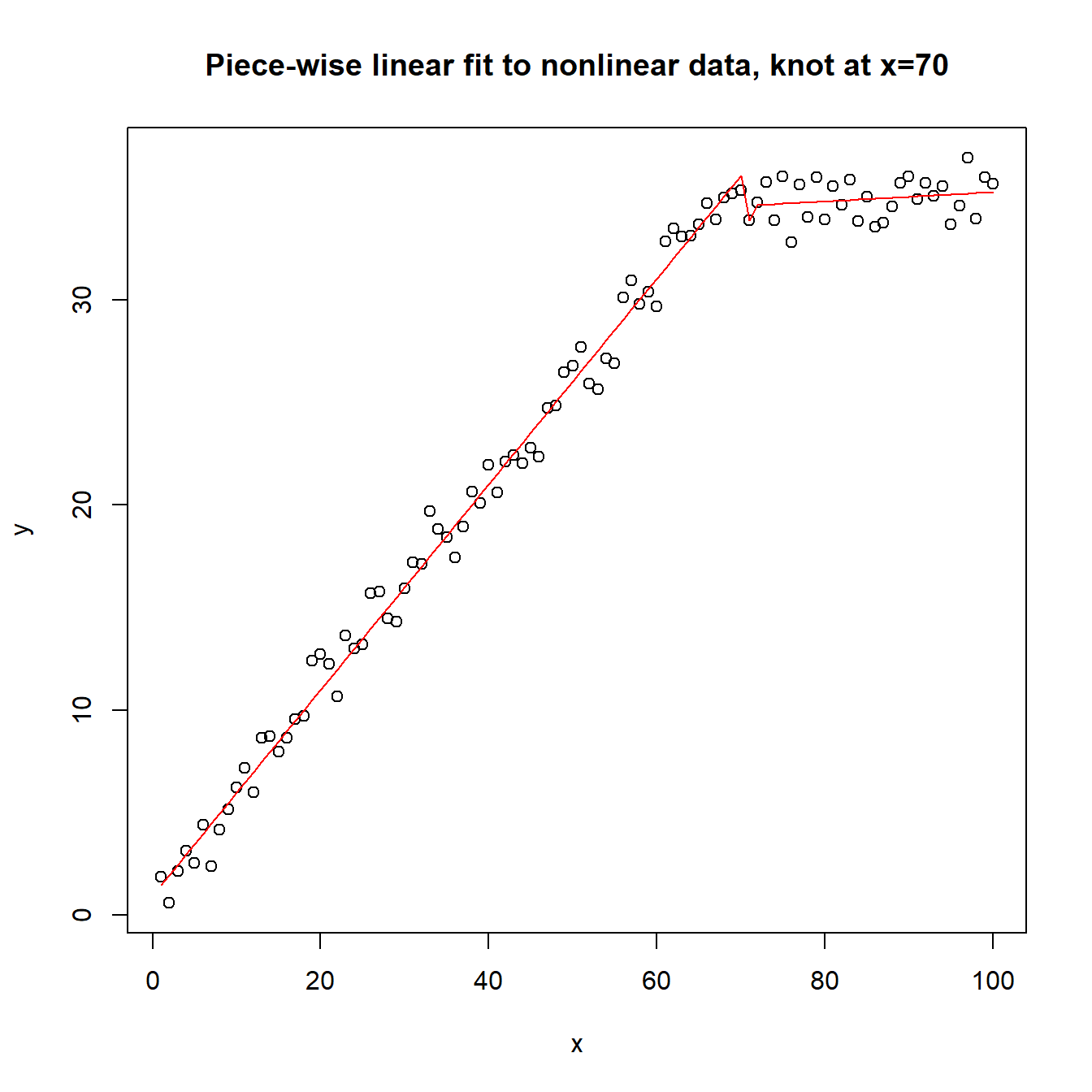Of course, this can be much more complicated, with multiple knots in the data, to model multiple changes in the underlying data generating fucntion

n <- 100 # number of data points
t <- seq(0,2*pi,,100)
a <- 3
b <- 2
c.norm <- rnorm(n)
amp <- 1

# generate data and calculate "y"
set.seed(1)
y2 <- a*sin(b*t)+c.norm*amp # Gaussian/normal error

# plot results
plot(t, y2, t="l", ylim=range(y2)*c(1,1.2), main="Sine curve with gaussian noise")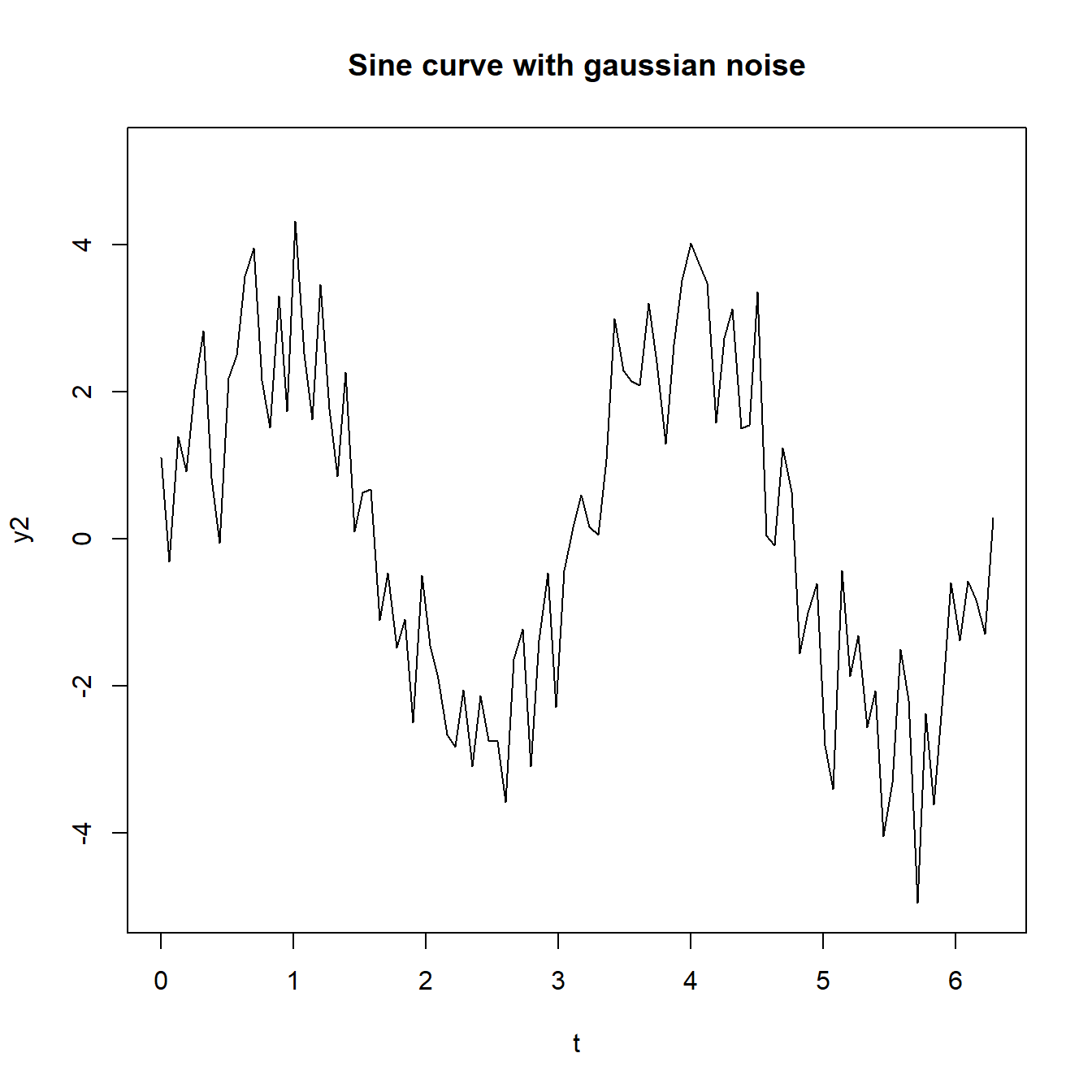plot(t, y2, t="l", ylim=range(y2)*c(1,1.2), main="Terrible linear model")

abline(lm(y2~t), col=3)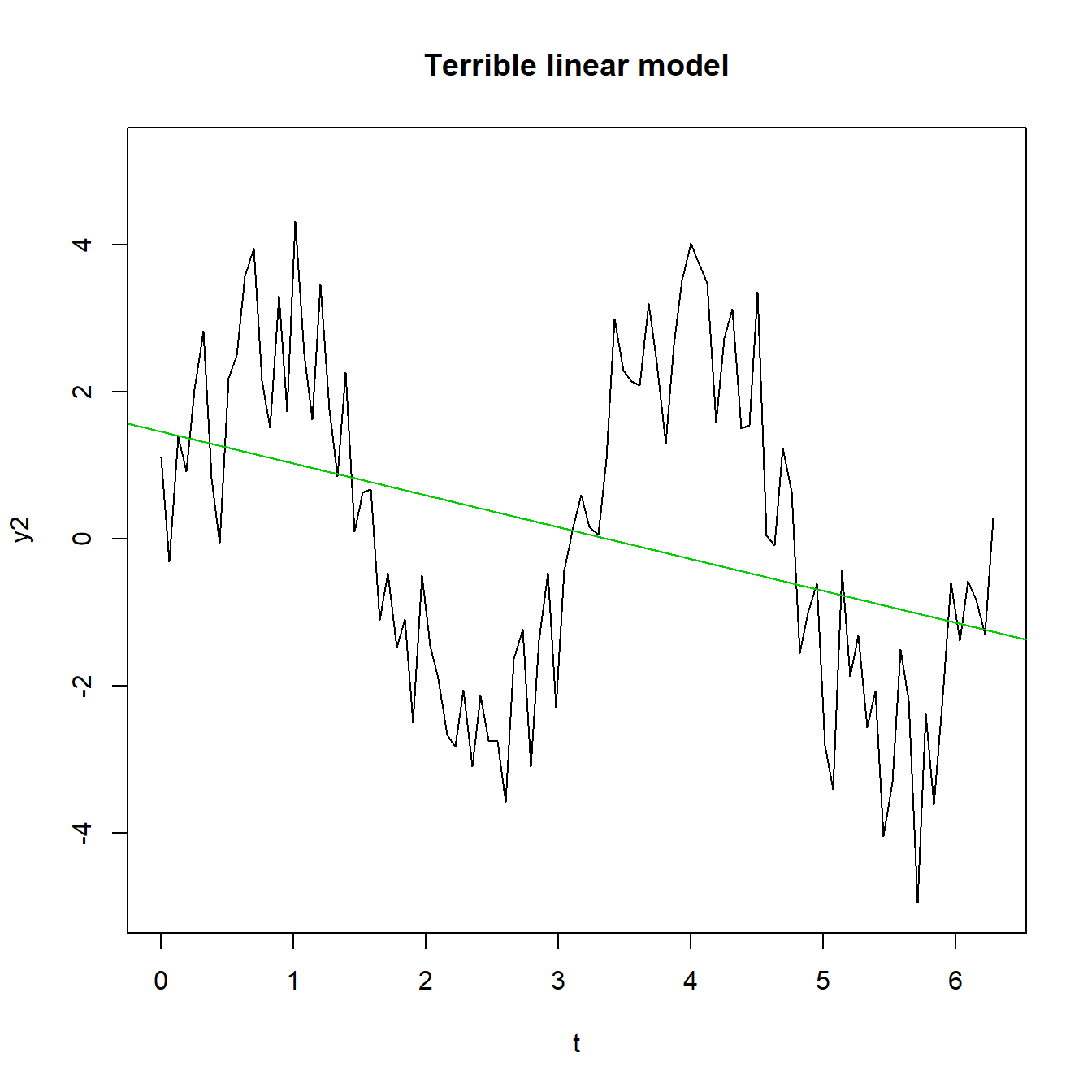Splines are often characterized by the number of knots used to build them

library(splines)
library(mgcv)
## Loading required package: nlme
## This is mgcv 1.8-28. For overview type 'help("mgcv-package")'.
plot(t, y2, t="l", ylim=range(y2)*c(1,1.2), main="Increasingly complex splines")
fit<-spline(y2,t)
lines(spline(t,y2, n = 4),col="darkgreen",lwd=2,type="l")
lines(spline(t,y2, n = 10),col="red",lwd=2,type="l")
lines(spline(t,y2, n = 20),col="blue",lwd=2,type="l")
lines(spline(t,y2, n = 30),col="orange",lwd=2,type="l")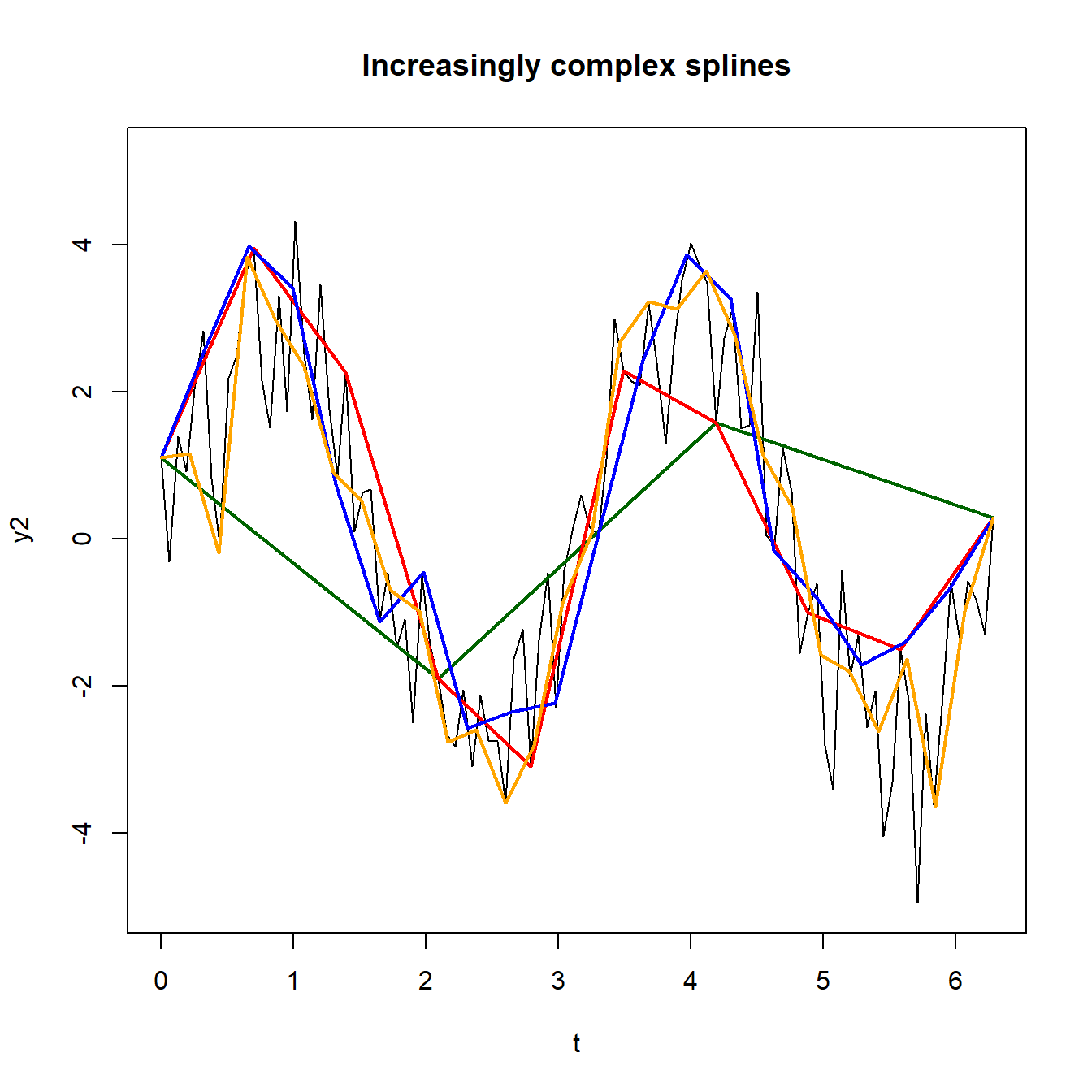## Cubic splines

The splines above were piecewise linear, we can also fit piecewise cubic splines, where at each knot a cubic polynomial is used. In the plot below, we can see that by increasing the number of knots, we better approximate the nonlinearities in the data.

plot(t, y2, t="l", ylim=range(y2)*c(1,1.2), main="Increasingly complex splines")

fit<-smooth.spline(t, y2, df=2)
fit5<-smooth.spline(t, y2, df=5)
fit10<-smooth.spline(t, y2, df=10)
lines(fit, col="red")
lines(fit5, col="green")
lines(fit10, col="blue")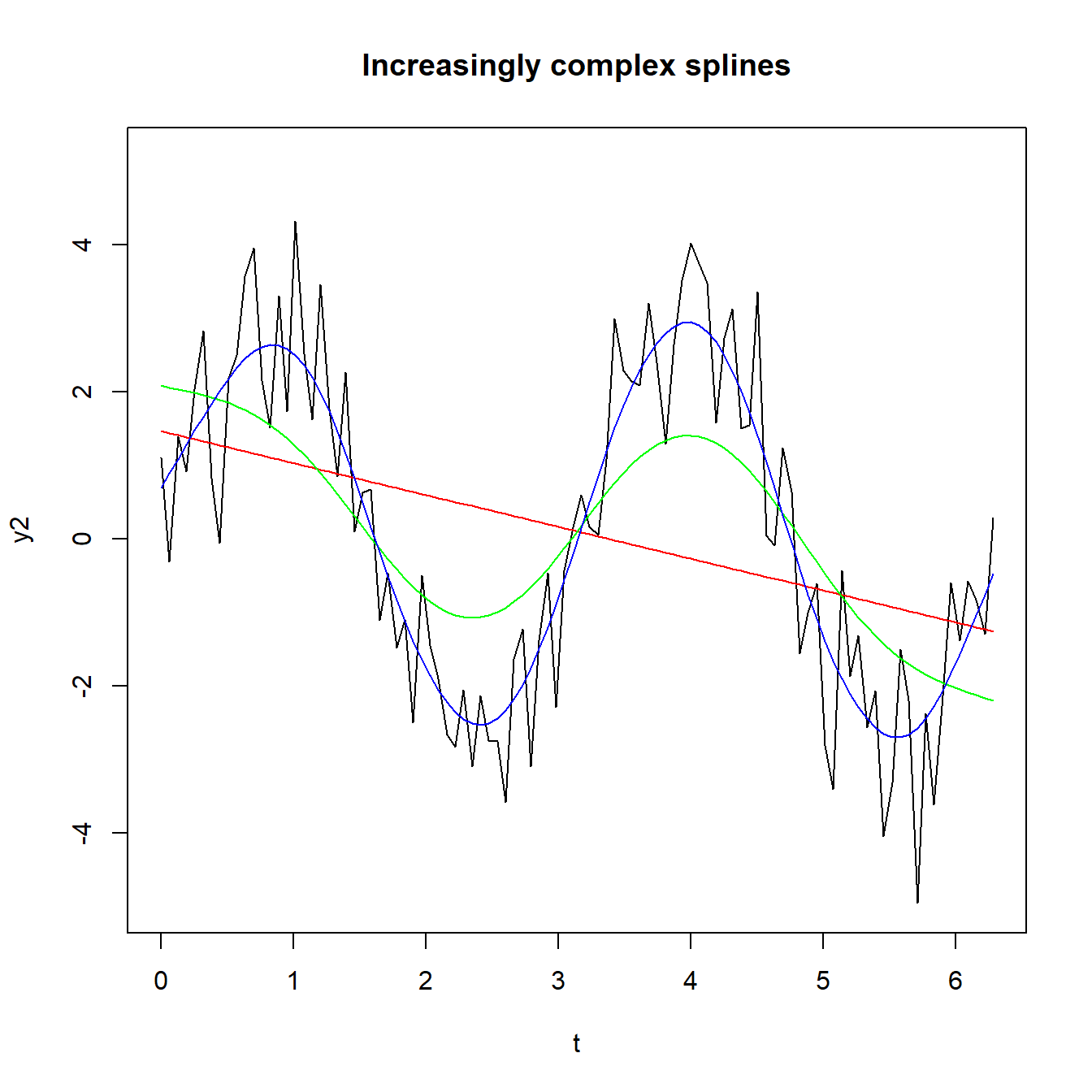## Regression splines

The generalized additive model (GAM) is a modeling framework that forms a linear predictor for a regression function through the combination of both smooth and linear terms.

The GAM model forms the linear predctor of a GLM as:

$E(y)= \beta_0 + f(x_1) + \beta_1 x_2$

where the $$f(x_1)$$ term is a regression spline of one of the variables. The models can be a mixture of linear and smooth terms.

library(mgcv) #one library for GAMs
library(ggplot2)
library(dplyr)
##
## Attaching package: 'dplyr'
## The following object is masked from 'package:nlme':
##
##     collapse
## The following objects are masked from 'package:stats':
##
##     filter, lag
## The following objects are masked from 'package:base':
##
##     intersect, setdiff, setequal, union
dat<-data.frame(y=y2, x=t)

gamfit<-gam(y~s(x), data=dat)
summary(gamfit)
##
## Family: gaussian
##
## Formula:
## y ~ s(x)
##
## Parametric coefficients:
##             Estimate Std. Error t value Pr(>|t|)
## (Intercept)  0.10273    0.09228   1.113    0.269
##
## Approximate significance of smooth terms:
##       edf Ref.df    F p-value
## s(x) 7.92  8.694 54.4  <2e-16 ***
## ---
## Signif. codes:  0 '***' 0.001 '**' 0.01 '*' 0.05 '.' 0.1 ' ' 1
##
## R-sq.(adj) =  0.829   Deviance explained = 84.3%
## GCV = 0.93506  Scale est. = 0.85165   n = 100
plot(gamfit)